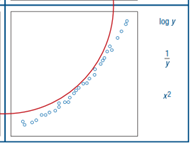# 2600 Scatter Plot Transformations

18 Questions | Total Attempts: 367Settings• 1.
When a scatter plot is non linear, the residual plot will be
• A.

Linear

• B.

Non-linear

• C.

Random

• D.

Patterned

• 2.
Which quadrant is displayed in the image above
• A.

• B.

• C.

• D.

• 3.
From this residual plot, we can tell the original scatter plot data is :
• A.

Linear

• B.

Non-linear

• C.

Random

• D.

Patterned

• 4.
From this residual plot, we can tell the original scatter plot data is :
• A.

Linear

• B.

Non-linear

• C.

Random

• D.

Patterned

• 5.
From this residual plot, we can tell the original scatter plot data is :
• A.

Linear

• B.

Non-linear

• C.

Random

• D.

Patterned

• 6.
From this residual plot, we can tell the original scatter plot data is :
• A.

Linear

• B.

Non-linear

• C.

Random

• D.

Patterned

• 7.
From this residual plot, we can tell the original scatter plot data is :
• A.

Linear

• B.

Non-linear

• C.

Random

• D.

Patterned

• 8.
From this residual plot, we can tell the original scatter plot data is :
• A.

Linear

• B.

Non-linear

• C.

Random

• D.

Patterned

• 9.
From this residual plot, we can tell the original scatter plot data is :
• A.

Linear

• B.

Non-linear

• C.

Random

• D.

Patterned

• 10.
From this residual plot, we can tell the original scatter plot data is :
• A.

Linear

• B.

Non-linear

• C.

Random

• D.

Patterned

• 11.
From this residual plot, we can tell the original scatter plot data is :
• A.

Linear

• B.

Non-linear

• C.

Random

• D.

Patterned

• 12.
From this residual plot, we can tell the original scatter plot data is :
• A.

Linear

• B.

Non-linear

• C.

Random

• D.

Patterned

• 13.
From this residual plot, we can tell the original scatter plot data is :
• A.

Linear

• B.

Non-linear

• C.

Random

• D.

Patterned

• 14.
From this residual plot, we can tell the original scatter plot data is :
• A.

Linear

• B.

Non-linear

• C.

Random

• D.

Patterned

• 15.
From this residual plot, we can tell the original scatter plot data is :
• A.

Linear

• B.

Non-linear

• C.

Random

• D.

Patterned

• 16.